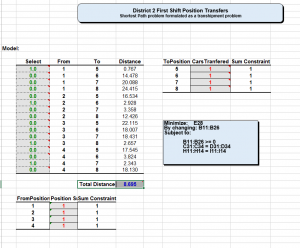In this tutorial I’m going to go through creating an optimization model in Excel. The next part of the tutorial will be scaling a small optimization program into a larger one by using the R programming language.

The problem I’m working on is: I have a list of police patrol car positions that I’ve put together, and I need to these positions to change every hour so we can minimize their response time when they respond to calls. These calls come from different parts of the city depending on the time of day, and day of week, that’s why we need to move them.

This type of problem is a variation of the Traveling Salesman Problem called the Transshipment problem and is commonly used to schedule shipments of cargo between a series of points.

To make the problem simpler, I’m going to isolate a small amount of the transfers, down to four patrol cars, transferring between two positions.  I’m going to skip a complete mathematical description of the transshipment problem for brevity here, and just talk through our particular problem. In Excel, we’re going to

• Minimize the total distance between the two sets of points by
• Changing a set of binary selection variables subject to:
• Each car is transferred to one, and only one point

This can be modeled in Excel, using the Excel Solver by arranging each set of possible points in a matrix, with the selection variable, the From points, the To points, and the distance between each possible combination of points. Since I have four From points, and four To points, I’ll have a matrix with sixteen rows with the different combinations. The From points are labeled one to four  and the To points are labeled five to eight. The Select variable in the left column will be utilized by the optimization engine to select the row, by putting a one or zero in the column. The Distance column defines the distance between the two points, we’re going to total that on the bottom if the Select column has a one in it.The total distances selected will define our optimization objective.

 Select From To Distance 1.0 1 5 0.767 0.0 1 6 14.478 0.0 1 7 20.088 0.0 1 8 24.415 0.0 2 5 16.534 1.0 2 6 2.928 0.0 2 7 3.358 0.0 2 8 12.426 0.0 3 5 22.115 0.0 3 6 18.007 0.0 3 7 18.431 1.0 3 8 2.657 0.0 4 5 17.545 0.0 4 6 3.824 1.0 4 7 2.343 0.0 4 8 18.130

To model the constraint that each from position will be assigned to one and only one to position, I’m going to set up two more tables in Excel. One table will track all the From assignments, and make sure that the assignments only add up to 1 for each from variable by using a sum constraint.

 FromPosition Position Sum Sum Constraint 1 1 1 2 1 1 3 1 1 4 1 1

The other table will track to To variables and use the same sum constraints to make sure that each To position only sums to 1.

 ToPosition Transferred Sum Constraint 5 1 1 6 1 1 7 1 1 8 1 1

Finally, I’m going to program this into the Excel solver. My Objective is to Minimize the total distance covered when I move the cars between their patrol positions.

 Total Distance 8.695

Then I need to put in a constraint to make sure all my binary selection variables stay positive, so there’s no funny business by the Excel solver to make the selection variables go negative, add my two table sum constraints, and finally, this is a linear program, so I’m going to make sure that Simplex LP is chosen for the Solving method.Press solve, and we’re done. Now that we’ve modeled this very simple model in Excel, the next time we’re going to transfer this problem to R, so we can scale it to more realistic schedules and situations.

The entire problem on Excel looks like this:Here’s the Excel file if you want to play with it:

transferLP

Part II of this tutorial, where we’ll turn the Excel model, into an R model is here:

Excel to R Transhipment Problem Optimization Tutorial Part II